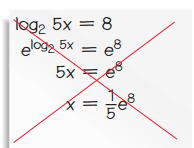#### MAT-HS.F-BF.05

 MAT-HS Targeted Standards(F) Concept: Function(BF) Domain: Building FunctionsCluster: Build new functions from existing functions MAT-HS.F-BF.05 Understand the inverse relationship between exponents and logarithms and use this relationship to solve problems involving logarithms and exponents.

• I can
• I can

• I can
• I can

• I can
• I can

• I can
• I can

## Alg II Solve Logarithmic Equations Proficiency Scale

 Score Description Sample Activity 4.0 (advanced) In addition to Score 3.0, the student demonstrates in-depth inferences and applications regarding more complex material that go beyond end of instruction expectations. Describe and correct the error in this work:3.5 In addition to Score 3.0 performance, the student demonstrates in-depth inferences and applications regarding the more complex content with partial success. 3.0 (proficient) The student can: solve a logarithmic equation and recognize an extraneous solution. The student exhibits no major errors or omissions. Solve the following logarithmic equation and check for extraneous solutions: log3(x2-30)=log3(x)    Solve the following logarithmic equation and check for extraneous solutions: 2log2(x)=log2(4)+log2(x-1) 2.5 The student demonstrates no major errors or omissions regarding the simpler details and processes (Score 2.0 content) and partial knowledge of the more complex ideas and processes (Score 3.0 content). 2.0 (progressing) There are no major errors or omissions regarding the simpler details and processes as the student can: recognize and recall specific terminology such as: Base Logarithm Exponentiate Condense Expand solve simple logarithmic equations. evaluate a logarithmic expression. use properties of logarithms to expand and condense an expression.  However, the student exhibits major errors or omissions regarding the more complex ideas and processes. Solve the following logarithmic equation for x: log10(100)=x 1.5 The student demonstrates partial knowledge of the simpler details and processes (Score 2.0 content) but exhibits major errors or omissions regarding the more complex ideas and procedures (Score 3.0 content). 1.0 (beginning) With help, the student demonstrates a partial understanding of some of the simpler details and processes (Score 2.0 content) and some of the more complex ideas and processes (Score 3.0 content). - 0.5 With help, the student demonstrates a partial understanding of some of the simpler details and processes (Score 2.0 content) but not the more complex ideas and processes (Score 3.0 content). 0.0 Even with help, the student demonstrates no understanding or skill. -

## Resources

 Web Vocab